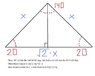# Special triangles problem

• Miike012

#### Miike012

I was reading how they found the ratios of the sides of a triangle.
It looks like they let the leg opposite of the 45 deg angle be length A and the corresponding 45 deg angle be length A aswell.. then they used the Pythagorean theorem to find the hyp.

Well I am curious if this can be done with any triangle? I have an attachment and I would like to know if I am correct? For my illustration I picked a 90.70.20 triangle at random.

#### Attachments

•MATH.jpg
27.5 KB · Views: 384

Miike012 said:
It looks like they let the leg opposite of the 45 deg angle be length A and the corresponding 45 deg angle be length A aswell.. then they used the Pythagorean theorem to find the hyp.

Well I am curious if this can be done with any triangle?

From the attachment, it seems that you assumed that all right triangles have its hyp to base ratio of $$x : x\ast\frac{\sqrt{2}}{2}$$. However, this is a special case for a 45, 45, 90 triangle.

It is because the two angles opposite the sides are equal. You can deduce this by the Pythagorean theorem:

If $$x^2 = a^2 + a^2$$ where x is the measure of the hypotenuse and a is the measure of the side

Then, $$x\ast\frac{\sqrt{2}}{2} = a$$

You will learn an easier way to determine the sides of a triangle in trigonometry. :)

Last edited:

Not simply any triangle, but a Right triangle. The pythagorean theorem works for Right triangles. For non-Right triangles, a modified formula works to relate the lengths of the sides.